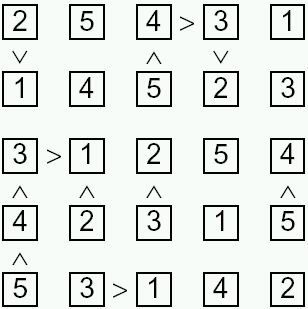Puzzle 1
Puzzle 2
Puzzle 3
Puzzle 4
Puzzle 5
Puzzle 6
Puzzle 7
Puzzle 8
Puzzle 9
Puzzle 10
Puzzle 11
Puzzle 12
Puzzle 13
Puzzle 14
Puzzle 15
Puzzle 16
Puzzle 17
Puzzle 18
Puzzle 19
Puzzle 20
Puzzle 21
Puzzle 22
Puzzle 23
Puzzle 24
Puzzle 25
Puzzle 26
Puzzle 27
Puzzle 28
Puzzle 29
Puzzle 30
Puzzle 31
Puzzle 32
Puzzle 33
Puzzle 34
Puzzle 35
Puzzle 36
Puzzle 37
Puzzle 38
Puzzle 39
Puzzle 40
Puzzle 41
Puzzle 42
Puzzle 43
Puzzle 44
Puzzle 45
Puzzle 46
Puzzle 47
Puzzle 48
Puzzle 49
Puzzle 50
Puzzle 51
Puzzle 52
Puzzle 53
Puzzle 54
Puzzle 55
Puzzle 56
Puzzle 57
Puzzle 58
Puzzle 59
Puzzle 60
Puzzle 61
Puzzle 62
Puzzle 63
Puzzle 64
Puzzle 65
Puzzle 66
Puzzle 67
Puzzle 68
Puzzle 69
Puzzle 70
Puzzle 71
Puzzle 72
Puzzle 73
Puzzle 74
Puzzle 75
Puzzle 76
Puzzle 77
Puzzle 78
Puzzle 79
Puzzle 80
Puzzle 81
Puzzle 82
Puzzle 83
Puzzle 84
Puzzle 85
Puzzle 86
Puzzle 87
Puzzle 88
Puzzle 89
Puzzle 90
Puzzle 91
Puzzle 92
Puzzle 93
Puzzle 94
Puzzle 95
Puzzle 96
Puzzle 97
Puzzle 98
Puzzle 99
Puzzle 100

# Futoshiki Puzzle

Welcome to one of the first Futoshiki Puzzles sites online!

Futoshiki is like sudoku except there is no box constraint. Instead, you have greater than and less than signs indicating the relationship between certain cells in the puzzle. This adds a delicious twist to the massively popular puzzle.Sample puzzle: Online Futoshiki Player

The standard futoshiki grid is either 5 x 5, 6 x 6 or 7 x 7 and requires you to place the numbers from 1 - 5 through 1 - 7 in the grid once per row and column and in obeyance with any inequalities shown.

In futoshiki there should be one valid solution per puzzle that can be reached through logic and clear thinking alone!

We can supply Futoshiki puzzles for publication in print-ready PDF format. Here is a Sample Futoshiki puzzle with solution on page two.

NEW! You can now download a PDF magazine of 100 standard Futoshiki puzzles laid out four per page with solutions: please take a look at Futoshiki Magazine

Or, if you prefer a physical book of puzzles, then take a look at the Futoshiki Puzzle Book page where you can choose to buy a book of 100 5x5, 6x6 or 7x7 futoshiki puzzles.

Here are some futoshiki strategy tips to help you solve the puzzles.

The first tip is to remember that you can only place a number once in each row and column - and with Futoshiki X, in each major diagonal. Therefore knock out numbers already placed in the regions that the cell you are trying to solve is in.

Remember also to use the essential rule from sudoku that each number must go once in each region - therefore if there is only one cell a 1 for instance can go in, you can place it there. Look especially for places that you can tell is either a '1' or '5' - this can help you start many futoshiki puzzles.

Also, remember that if two cells contain the same two numbers alone as possibilities, you can eliminate these from other cells in that region. Imagine the region looks like this:
12 | 12 | 123 | 345 | 345 we know that this must be: 12 | 12 | 3 | 45 | 45

Good luck solving the puzzles - remember to have fun!

# Futoshiki Equality

Another variant of futoshiki / hutosiki that you might have seen is Futoshiki Equality. This version of the puzzle also uses the equality relationship in addition to the less than and greater than sign, to show that some cells contain the same values (clearly across diagonals as a number can only be placed once per row and column). Here is a sample Equality Futoshiki - another fun variant of this increasingly popular puzzle!

You can play Thousands of Puzzles Online across over twenty different puzzle types.

If you want puzzles for print publication, whether futoshiki or one of many other common or unusual puzzles, check our our Puzzle Portfolio.